# Egham-Reading-London Arithmetic Statistics Seminar

## Programme for Thursday 22nd April 2021 (online)

09:30 – 10:30 Igor Wigman (King’s College London): On the distribution of lattice points on hyperbolic circles
10:45 – 11:45 James Maynard (Oxford): Simultaneous small fractional parts of polynomials
12:00 – 13:00 Gautami Bhowmik (Lille): Some Conditional Bounds on Siegel zeros

Time zone is GMT+1.

## Abstracts

Bhowmik: A Landau-Siegel zero is a possible counter-example to the Riemann Hypothesis for Dirichlet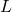-functions. It is known that such a zero, if it does exist, would be very close to 1. Almost all improvements of classical results on this distance requires some additional conditions. We will discuss some of these. In particular we will assume certain weak Goldbach conjectures to obtain refinements. Finally we could suggest some possible lines of further work.
This is joint work with Karin Halupczok.

Maynard: Givenreal numbers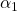, … ,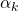, how well can you simultaneously approximate these real numbers with squares? More specifically, how small can you make all the fractional parts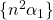, … ,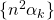using integers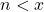? Classical work of Schmidt shows that there is an integersuch that all of these fractional parts are at most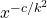(for some constant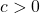), uniformly for every choice of. I’ll present some recent work which improves this bound to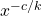, which is optimal up to the value of the constantand similar results for more general polynomials instead of the squares. The improvement comes from using a fun blend of additive combinatorics and geometry of numbers.

Wigman: This talk is based on a joint work with D. Chatzakos, P. Kurlberg and S. Lester.
We study the fine distribution of lattice points lying on expanding circles in the hyperbolic plane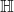. The angles of lattice points arising from the orbit of the modular group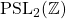, and lying on hyperbolic circles, are shown to be equidistributed for generic radii. However, the angles fail to equidistribute on a thin set of exceptional radii, even in the presence of growing multiplicity. Surprisingly, the distribution of angles on hyperbolic circles turns out to be related to the angular distribution of-lattice points (with certain parity conditions) lying on circles in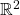, along a thin subsequence of radii. A notable difference is that measures in the hyperbolic setting can break symmetry — on very thin subsequences they are not invariant under rotation by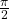, unlike the Euclidean setting where all measures have this invariance property.

## ERLASS organisers

Steve Lester (King’s College London)
Rachel Newton (Reading)
Yiannis Petridis (UCL)
Ian Petrow (UCL)
Martin Widmer (Royal Holloway)

Local organiser: Rachel Newton (r.d.newton@reading.ac.uk)

This is the third meeting of the Egham-Reading-London Arithmetic Statistics Seminar.

Sponsor: ERLASS is funded by a London Mathematical Society Scheme 3 grant (31902).